# Ten Open problems in Approximation Algorithms Dead problems

• Slides: 41Ten Open problems in Approximation Algorithms: Dead problems society Guy Kortsarz 12 of June 2020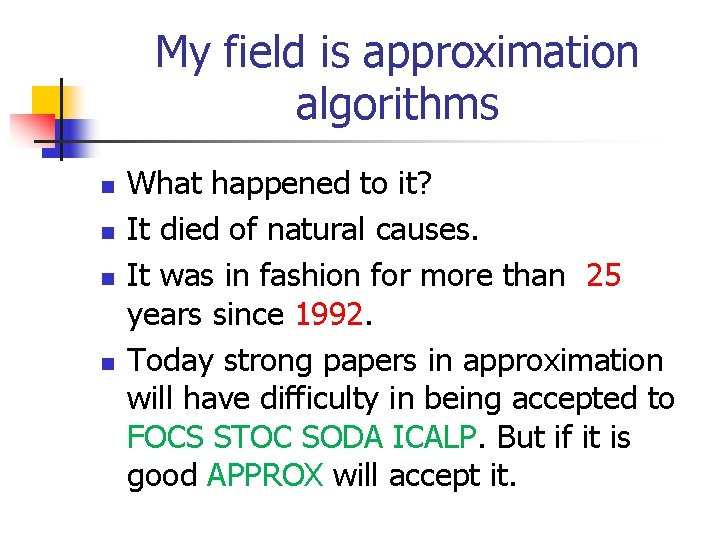My field is approximation algorithms n n What happened to it? It died of natural causes. It was in fashion for more than 25 years since 1992. Today strong papers in approximation will have difficulty in being accepted to FOCS STOC SODA ICALP. But if it is good APPROX will accept it.Now other fields are popular n n n Streaming Machine Learning Computer Security Quantom Algorithms. Algorithm Game theory (maybe it is over the hill as well) Computational biology.David P. Williamson With Shmoys: Open problems n n They give 10 problems some quite hard and open for a long time. Most of these problems I have no idea about and so I will not present them. I will take the very few that I somewhat know and say a word. I will not define the problem (famous).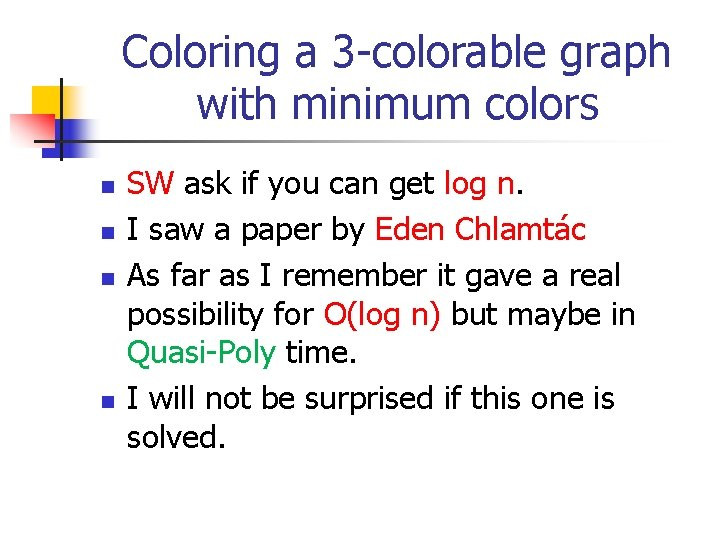Coloring a 3 -colorable graph with minimum colors n n SW ask if you can get log n. I saw a paper by Eden Chlamtác As far as I remember it gave a real possibility for O(log n) but maybe in Quasi-Poly time. I will not be surprised if this one is solved.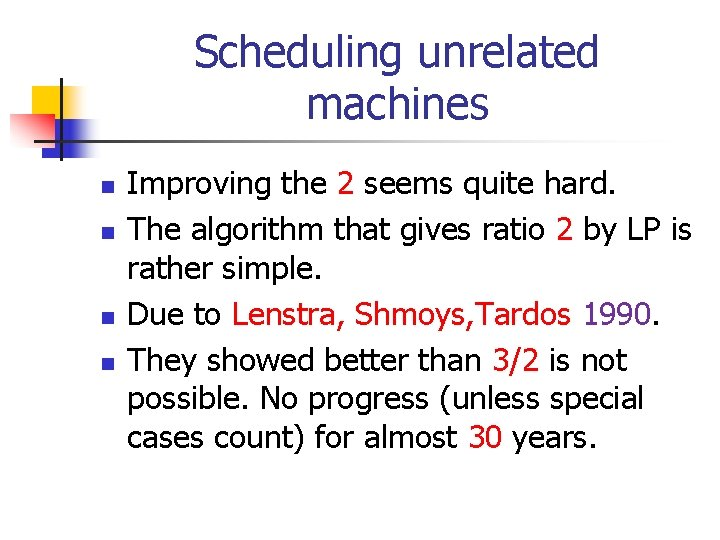Scheduling unrelated machines n n Improving the 2 seems quite hard. The algorithm that gives ratio 2 by LP is rather simple. Due to Lenstra, Shmoys, Tardos 1990. They showed better than 3/2 is not possible. No progress (unless special cases count) for almost 30 years.Minimum Steiner Forest n n A primal-dual 2 -approximation algorithm (Agrawal, Klein, Ravi 1995; see also GW 1995). This question is breaking the 2. May not be possible. One of the most basic open problems.Survivable network design n AKA: Steiner Network. Jain gave a somewhat surprising (maybe for me) ratio 2. Is this the best? Can it be done by a combinatorial algorithm? Seems very hard to answer.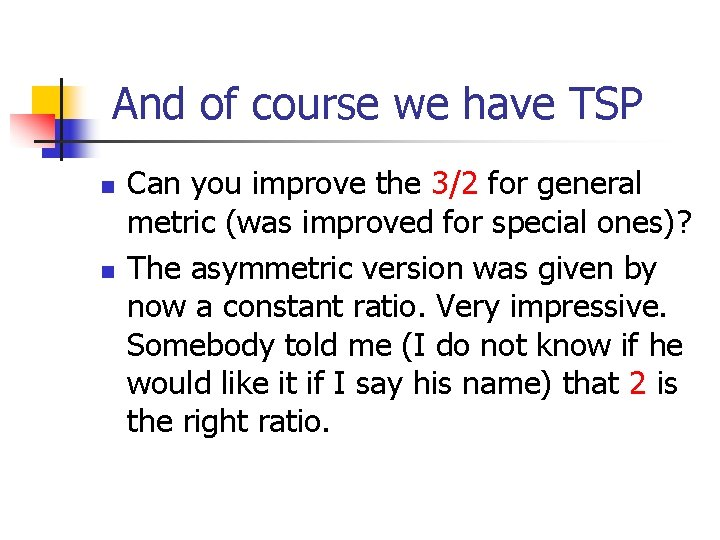And of course we have TSP n n Can you improve the 3/2 for general metric (was improved for special ones)? The asymmetric version was given by now a constant ratio. Very impressive. Somebody told me (I do not know if he would like it if I say his name) that 2 is the right ratio.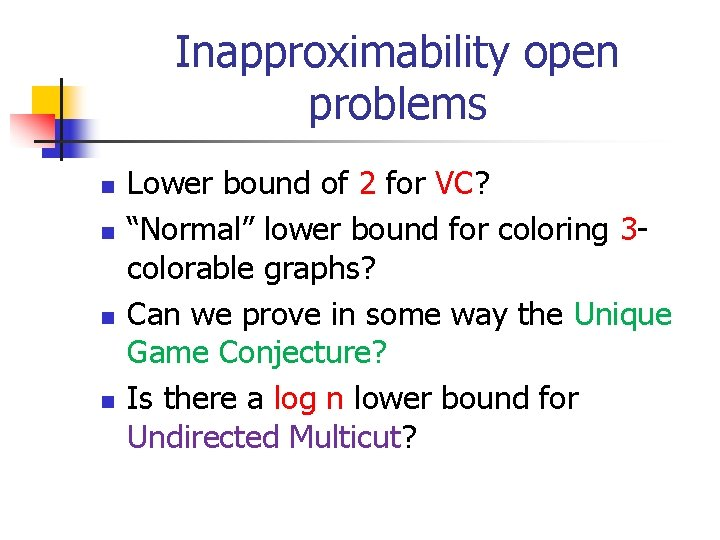Inapproximability open problems n n Lower bound of 2 for VC? “Normal” lower bound for coloring 3 colorable graphs? Can we prove in some way the Unique Game Conjecture? Is there a log n lower bound for Undirected Multicut?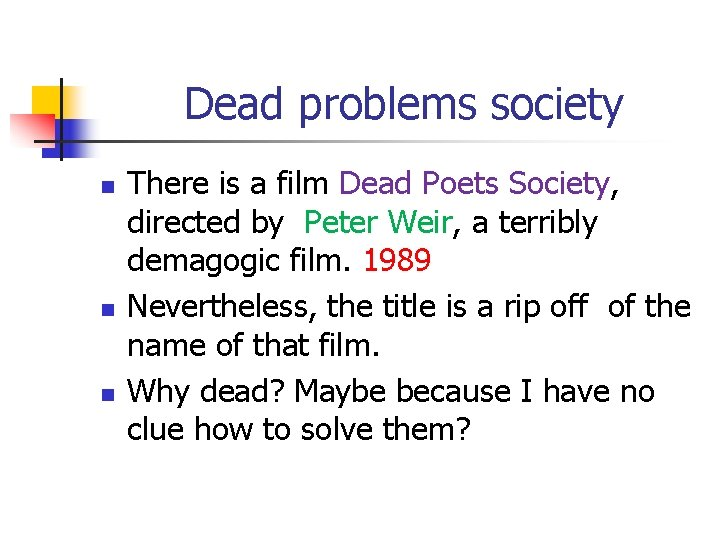Dead problems society n n n There is a film Dead Poets Society, directed by Peter Weir, a terribly demagogic film. 1989 Nevertheless, the title is a rip off of the name of that film. Why dead? Maybe because I have no clue how to solve them?Problem 1: Approximating Multicommodity Buy-At-Bulk n n State of affairs: O(log 3 n) ratio for polynomial demands Due to Kortsarz, Nutov, TCS, 2011. For general demands best known O(log 4 n) by Chekuri, Hajiaghayi, Kortsarz, Salavatipour. In SICOMPThe best known lower bound n n (sqrt{log n}) Matthew Andrews: Hardness of Buy-at-Bulk Network Design. FOCS 2004. There is no progress in upper or lower bounds, as far as I know, for a very long time. Please solve it next Friday.Buy at Bulk with protection n n See a paper by Chandra Chekuri. For this problem there are many hard open problems. I do not know how to solve them. Maybe solve it between Breakfast to Lunch.Problem 2: FPT-hardness for Clique n n Is the Clique problem completely FPT inapproximable under the ETH? Complete FPT inapproximability is known for Set Cover. See paper by Karthik, Laekhanukit, Manurangsi. Known to be true under GAP-ETH. Should be one of the easier problems.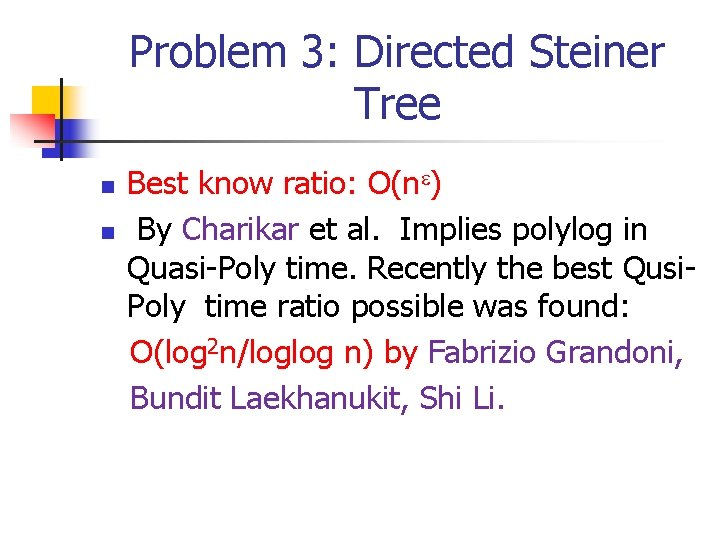Problem 3: Directed Steiner Tree n n Best know ratio: O(n ) By Charikar et al. Implies polylog in Quasi-Poly time. Recently the best Qusi. Poly time ratio possible was found: O(log 2 n/loglog n) by Fabrizio Grandoni, Bundit Laekhanukit, Shi Li.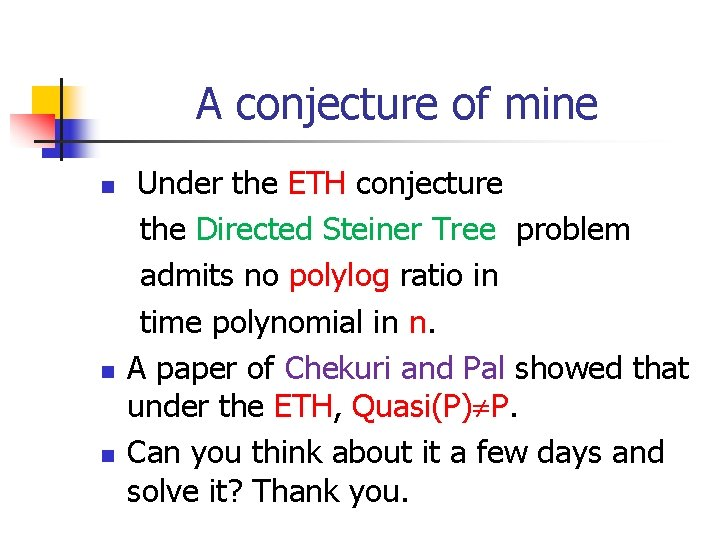A conjecture of mine n n n Under the ETH conjecture the Directed Steiner Tree problem admits no polylog ratio in time polynomial in n. A paper of Chekuri and Pal showed that under the ETH, Quasi(P) P. Can you think about it a few days and solve it? Thank you.Problem 4: Shallow Light Trees n n Shallow light Steiner trees: Given a graph with length 1 on the edges and arbitrary positive costs c(e) on the edges, a root r, and a height bound h. And a collection of terminals. Question: Find a Steiner tree of height at most h and among them minimum cost (the height bound is hard const’)The ratio n n n A ratio similar to the one of Directed Steiner Tree can be derived. Kortsarz, Peleg Known since 1997. In fact if you can not have height even h+1, as hard as DST (easy but not published result). My open question: is there bicriteria (O(1), polylog n) approximation?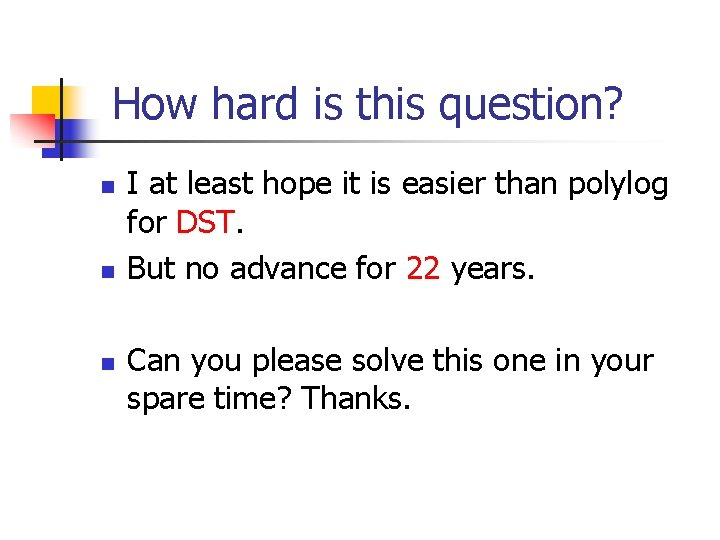How hard is this question? n n n I at least hope it is easier than polylog for DST. But no advance for 22 years. Can you please solve this one in your spare time? Thanks.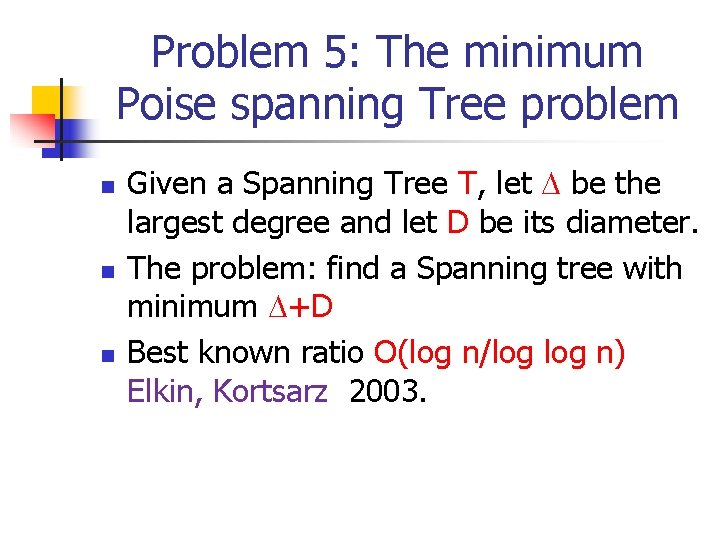Problem 5: The minimum Poise spanning Tree problem n n n Given a Spanning Tree T, let be the largest degree and let D be its diameter. The problem: find a Spanning tree with minimum +D Best known ratio O(log n/log n) Elkin, Kortsarz 2003.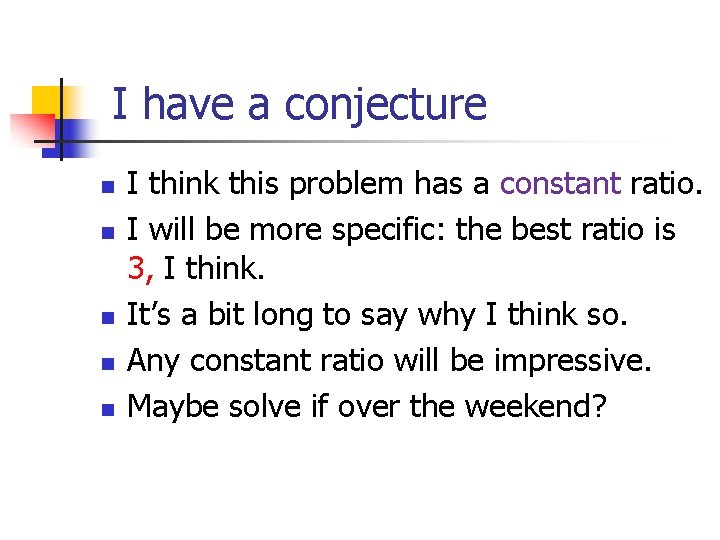I have a conjecture n n n I think this problem has a constant ratio. I will be more specific: the best ratio is 3, I think. It’s a bit long to say why I think so. Any constant ratio will be impressive. Maybe solve if over the weekend?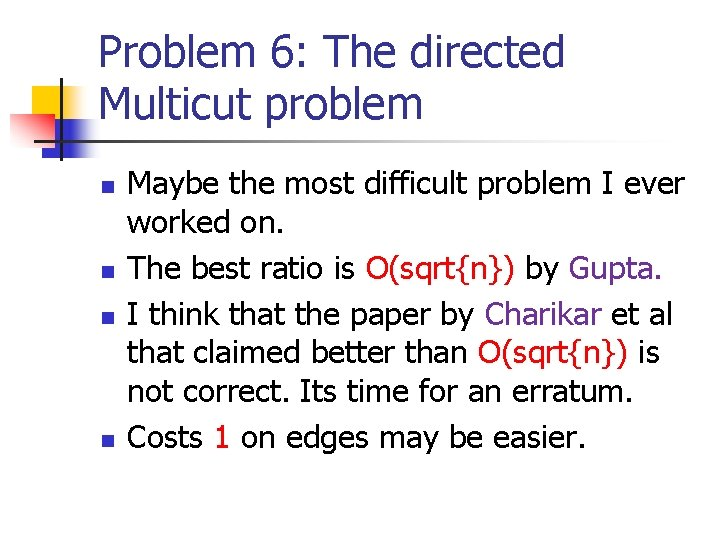Problem 6: The directed Multicut problem n n Maybe the most difficult problem I ever worked on. The best ratio is O(sqrt{n}) by Gupta. I think that the paper by Charikar et al that claimed better than O(sqrt{n}) is not correct. Its time for an erratum. Costs 1 on edges may be easier.What is known n n O(opt) ratio. Anupam Gupta. A paper of mine gives a O(n 2/3/opt 1/3) ratio for unit costs. Nobody knows it. This means that if opt (sqrt{n}) we can break the sqrt{n}. So assume opt= (sqrt{n}), and improve the ratio. I will be happy if anyone gives better than O(sqrt{n})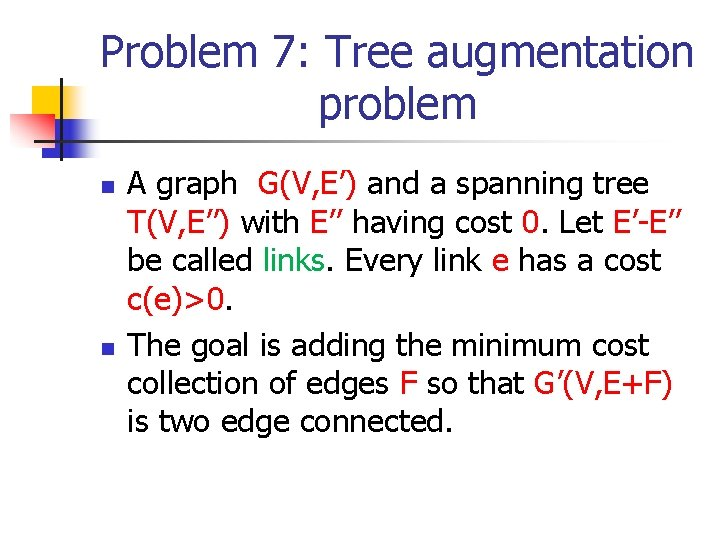Problem 7: Tree augmentation problem n n A graph G(V, E’) and a spanning tree T(V, E’’) with E’’ having cost 0. Let E’-E’’ be called links. Every link e has a cost c(e)>0. The goal is adding the minimum cost collection of edges F so that G’(V, E+F) is two edge connected.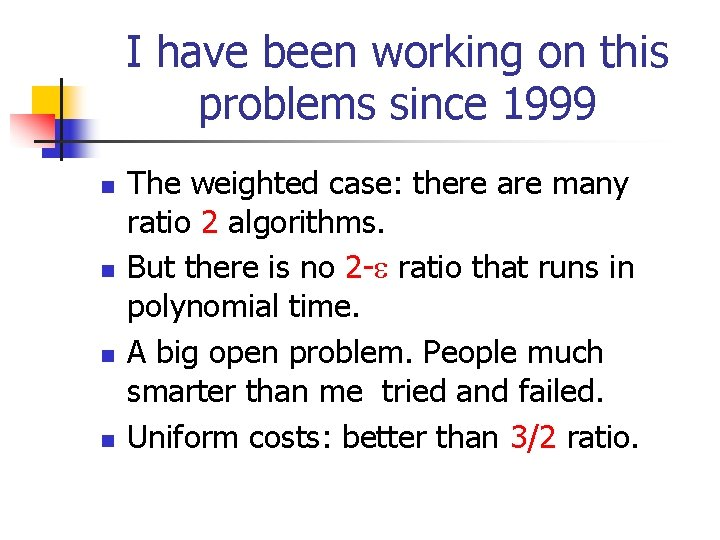I have been working on this problems since 1999 n n The weighted case: there are many ratio 2 algorithms. But there is no 2 - ratio that runs in polynomial time. A big open problem. People much smarter than me tried and failed. Uniform costs: better than 3/2 ratio.One of the problems I worked most on n So far we failed badly. If the maximum edge cost is a constant there are for the costs version better than 2 ratio. But non of the methods used for uniform costs or bounded maximum weight seems even close to the general problem. In my opinion central open problem. Solve it tomorrow.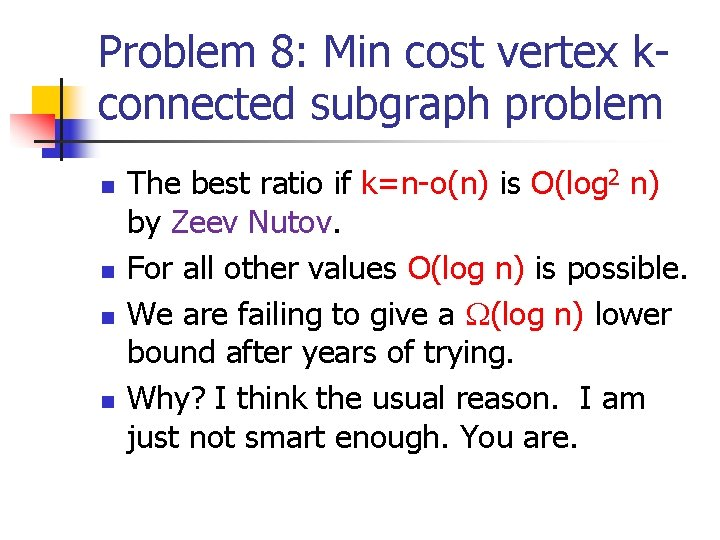Problem 8: Min cost vertex kconnected subgraph problem n n The best ratio if k=n-o(n) is O(log 2 n) by Zeev Nutov. For all other values O(log n) is possible. We are failing to give a (log n) lower bound after years of trying. Why? I think the usual reason. I am just not smart enough. You are.Problem 9: Many problems on Group Steiner n n n Is O(log 2 n/loglog n) possible in poly time on trees? The integrality gap can not be more than that. Many tried this one, some smart people, and failed. Is there a ratio for general graph that equals the ratio for trees? Do we need FRT to solve the general case?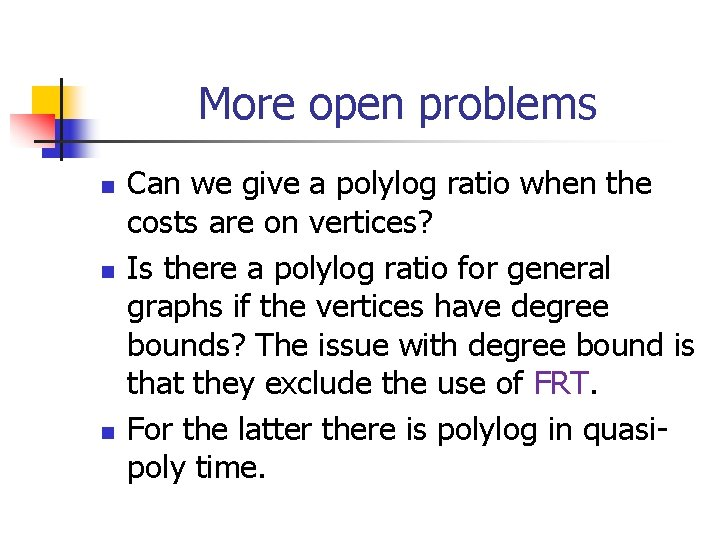More open problems n n n Can we give a polylog ratio when the costs are on vertices? Is there a polylog ratio for general graphs if the vertices have degree bounds? The issue with degree bound is that they exclude the use of FRT. For the latter there is polylog in quasipoly time.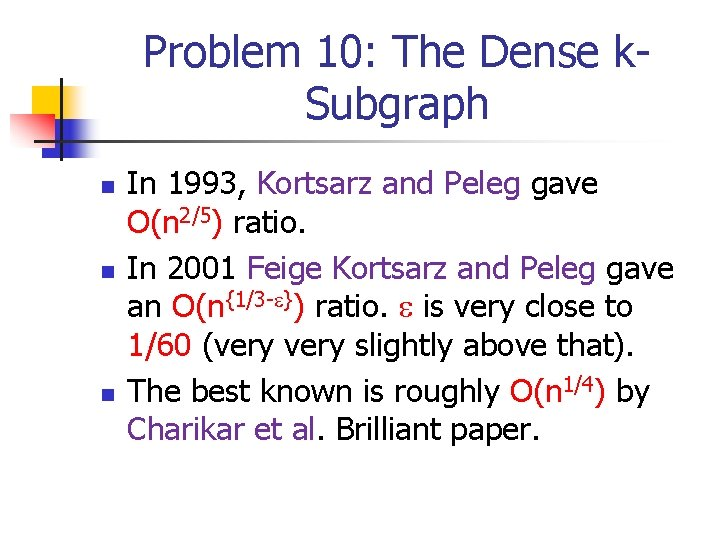Problem 10: The Dense k. Subgraph n n n In 1993, Kortsarz and Peleg gave O(n 2/5) ratio. In 2001 Feige Kortsarz and Peleg gave an O(n{1/3 - }) ratio. is very close to 1/60 (very slightly above that). The best known is roughly O(n 1/4) by Charikar et al. Brilliant paper.The question is n n n Is there any hardness under P NP? So far we know lower bound under the ETH and under random assumptions. I am certain that the ratio by Charikar et al is the best. This is related to the so called “Log Density Conjecture”. Maybe solve it next Monday?Are my ten open problems any good? n n I think they are. Approximation Algorithms reached a dead end. The important problems left are very hard. I advise that students will not go for a Ph. D in Approximation Algorithms.What to do n n Maybe do not do a Ph. D. and go to the industry? Theory is a small field. Few come to theory conferences. The field does not have a lot of grant money. Just do not go to theory (either applied fields or the industry).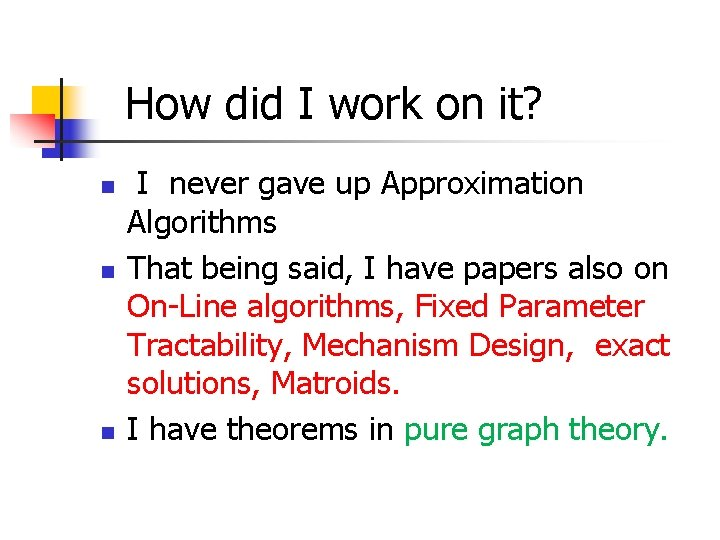How did I work on it? n n n I never gave up Approximation Algorithms That being said, I have papers also on On-Line algorithms, Fixed Parameter Tractability, Mechanism Design, exact solutions, Matroids. I have theorems in pure graph theory.Theoretical Computer Science should change n n n The time in which people like me are doing papers in theoretical computer science has to end. Theoterical computer science should becomes like math Only true geniuses should work on it, and in general not many should work on it.My first paper was in 1992 n n In 2022 it will be 30 years from my first paper I was sick for 8 of these years so I actually worked 23 years. It is very tempting to leave research at that time. I do not have the same energy.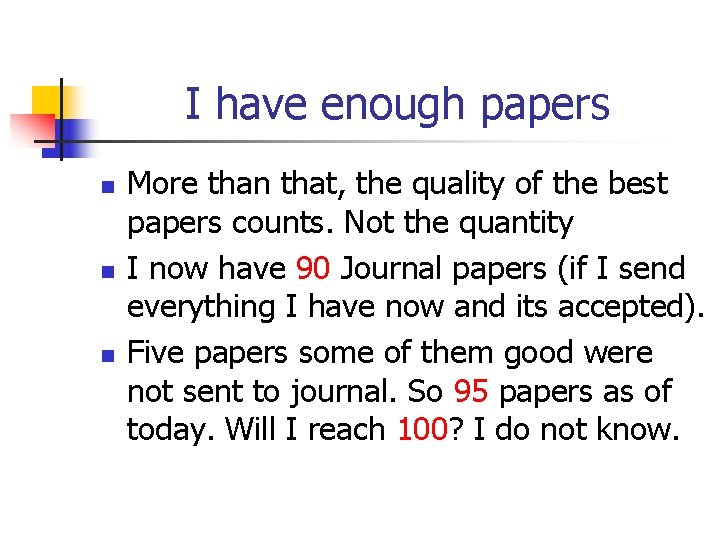I have enough papers n n n More than that, the quality of the best papers counts. Not the quantity I now have 90 Journal papers (if I send everything I have now and its accepted). Five papers some of them good were not sent to journal. So 95 papers as of today. Will I reach 100? I do not know.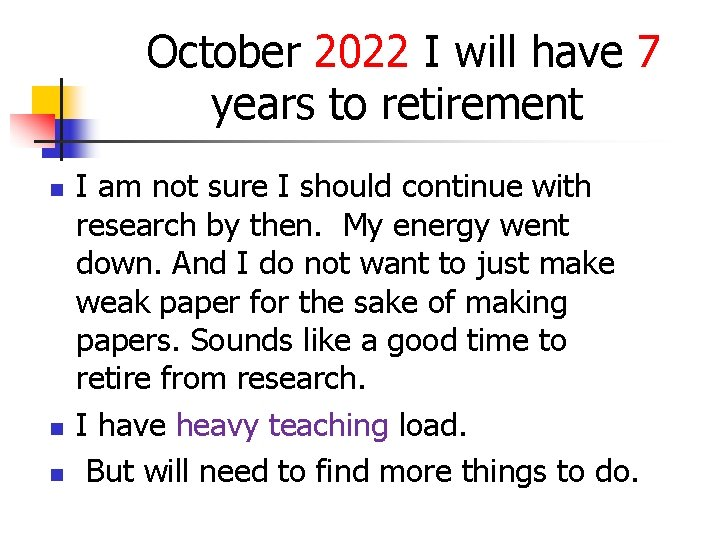October 2022 I will have 7 years to retirement n n n I am not sure I should continue with research by then. My energy went down. And I do not want to just make weak paper for the sake of making papers. Sounds like a good time to retire from research. I have heavy teaching load. But will need to find more things to do.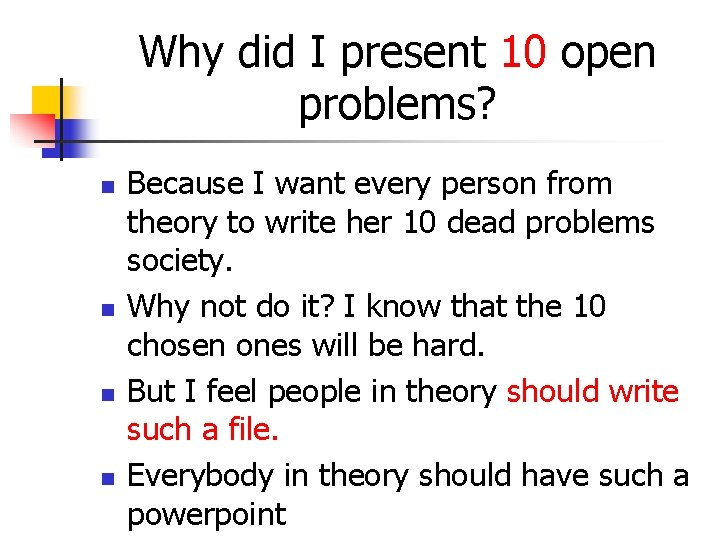Why did I present 10 open problems? n n Because I want every person from theory to write her 10 dead problems society. Why not do it? I know that the 10 chosen ones will be hard. But I feel people in theory should write such a file. Everybody in theory should have such a powerpointPlease do so. Its fun! n n I will be happy to hear your 10 favorite open problems. If it is in theory I am likely to understand Do not write on the famous open problems. Just those you worked a lot on. Looking forward to you Dead Problems Society!